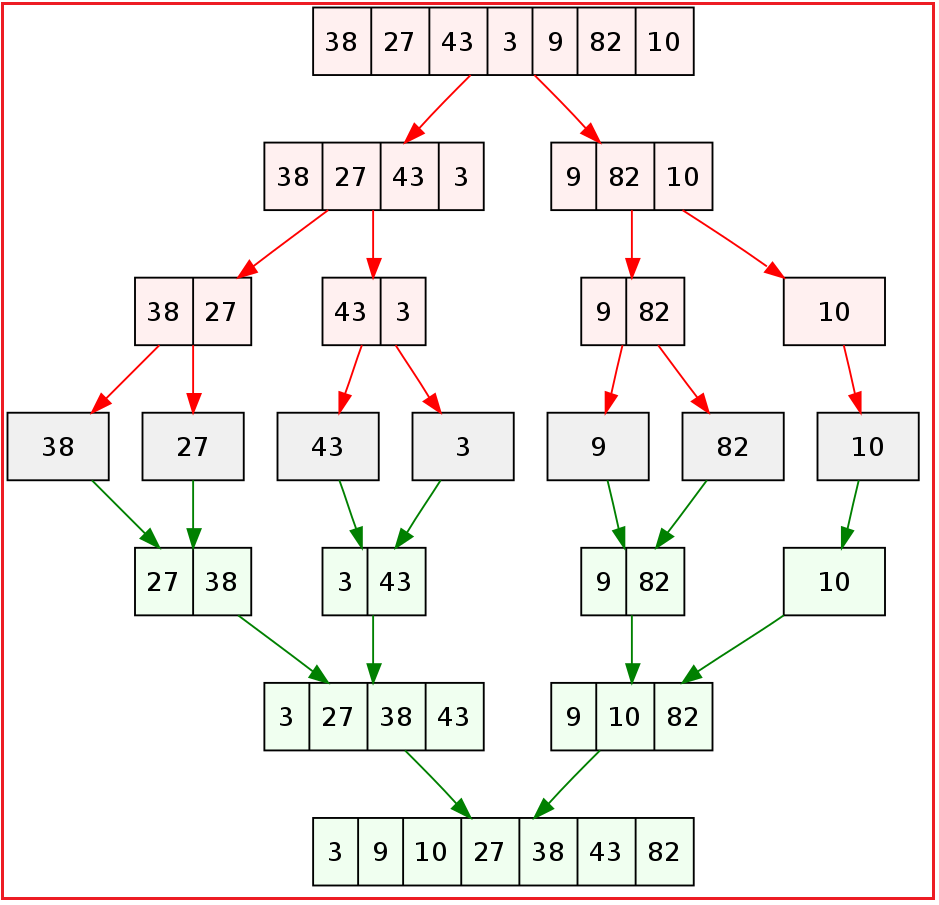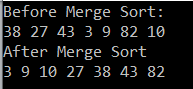# Merge Sort in C#

## Merge Sort in C# with Example

In this article, I am going to discuss the Merge Sort in C# with Example. Please read our previous article before proceeding to this article where we discussed the Bubble Sort Algorithm in C# with example. The Merge Sort Algorithm in C# is a sorting algorithm and used by many programmers in real-time applications.

##### The Merge Sort Algorithm:

The Merge Sort Algorithm is a comparison-based sorting algorithm that is based on the divide and conquers approach.

##### What is the Divide and Conquer Approach?

The divide and conquer approach means it will divide the input array into sub-arrays and then further it divide the sub-arrays into sub-arrays until each sub-array contains a single element. Then each sub-arrays merge together in such a way that it will form a single sorted array.

##### How does the Merge Sort Algorithm work?

The Merge Sort Algorithm works as follows:

1. Divide the unsorted array into n sub-arrays, each array containing a single element.
2. Repeatedly merge the sub-arrays to produce a new sorted array until there is only 1 array remaining.

Note: The idea behind the merge sort is that it is going to merge two sorted arrays.

Let us understand this with an example. We have an input integer array having the elements as 38, 27, 43, 3, 9, 82, and 10. Let’s have a look at the following diagram which shows how the merge sort is working.Let us understand the step-by-step procedure to understand the merge with the example shown in the above image.

##### Step1: Dividing

In step1, we need to divide the array into two sub-arrays. Here the first sub-array will contain four elements (such as 38, 27, 43, and 3) and the other sub-array will contain 3 elements such as (9, 82, and 10).

The first subarray which contains 38, 27, 43, and 3 is further divided into two subarrays (38, 27) and (43, 3). The subarray which contains (38, 27) is further divided into (28) and (27). Similarly, the subarray which contains (43, 3) will be divided into subarrays (43) and (3). At this point, the division will stop as each sub-array contains a single element.

The second subarray which contains 9, 82, and 10 is further divided into two subarrays. One sub-array with two elements i.e. 9 and 82 and the other sub-array with a single element i.e. 10. Further, the subarray which contains (9, 82) is divided into (9) and (82). At this point as each sub-array contains a single element so the division will stop here.

##### Step2: Merge

At the end of the step1, we have seven subarrays and each one containing a single element in it. In step2, we need to merge the sub-arrays. The Subarrays (38) and (27) will merge together to form a sorted subarray i.e. (27, 38). Similarly, the other subarrays (43) and (3) will merge together to form a sorted subarray (3, 43) and the subarrays (9) and (82) will merge together to form a sorted subarray (9, 82). In this step, we will keep 10 as it is.

Now the sub-arrays (27, 38) and (43, 3) will merge together to form a sorted subarray i.e. (3, 27, 38, and 43). Similarly, the other two subarrays (9, 82) and 10 merge together to form a sorted subarray (i.e. 9, 10, and 82).

Now, we have two sub-arrays i.e. (3, 27, 38, and 43) and (9, 10, and 82) will merge together to finally produce the sorted array such as (3, 9, 10, 27, 38, 43, and 82).

##### Program to Implement Merge Sort in C#:
```using System;
namespace LogicalPrograms
{
class Program
{
static public void MergeMethod(int[] numbers, int left, int mid, int right)
{
int[] temp = new int;
int i, left_end, num_elements, tmp_pos;
left_end = (mid - 1);
tmp_pos = left;
num_elements = (right - left + 1);
while ((left <= left_end) && (mid <= right))
{
if (numbers[left] <= numbers[mid])
temp[tmp_pos++] = numbers[left++];
else
temp[tmp_pos++] = numbers[mid++];
}
while (left <= left_end)
temp[tmp_pos++] = numbers[left++];
while (mid <= right)
temp[tmp_pos++] = numbers[mid++];
for (i = 0; i < num_elements; i++)
{
numbers[right] = temp[right];
right--;
}
}
static public void SortMethod(int[] numbers, int left, int right)
{
int mid;
if (right > left)
{
mid = (right + left) / 2;
SortMethod(numbers, left, mid);
SortMethod(numbers, (mid + 1), right);
MergeMethod(numbers, left, (mid + 1), right);
}
}
static void Main(string[] args)
{
int[] numbers = { 38, 27, 43, 3, 9, 82, 10 };
int len = numbers.Length;
Console.WriteLine("Before Merge Sort:");
foreach(int item in numbers)
{
Console.Write(item + " ");
}
Console.WriteLine();

Console.WriteLine("After Merge Sort");
SortMethod(numbers, 0, len - 1);

foreach (int item in numbers)
{
Console.Write(item + " ");
}
}
}
}
```

Output:##### Time Complexity of Merge Sort in C#:

The Merge Sort Algorithm is a recursive algorithm. The array of size N is divided into the maximum of logN parts, and the merging of all the subarrays into a single array takes O(N) time. Hence in all three cases (worst, average, best), the time complexity of Merge sort is O(nlogn).

##### Algorithm for C# Merge Sort:

To sort the array A[1 .. n], make the initial call to the procedure MERGE-SORT (A, 1, n).

MERGE-SORT (A, p, r)

1. IF p < r // Check for base case
2. THEN q = FLOOR[(p + r)/2] // Divide step
3. MERGE (A, p, q) // Conquer step.
4. MERGE (A, q + 1, r) // Conquer step.
5. 5. MERGE (A, p, q, r) // Conquer step.

In the next article, I am going to discuss the Insertion Sort in C# with examples. Here, in this article, I try to explain the Merge Sort in C# with example. I hope now you understood how Merge Sort Algorithm Works in C# to sort an unsorted array.

### 1 thought on “Merge Sort in C#”

1.